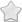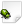OPEN  Crash report #1597  -  [34AD08AA] ??()
Posted Dec 30, 2021 - updated Dec 30, 2021   Shortlink: http://arx.vg/1597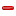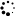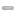0 Votes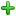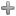Issue details
• Type of issue
Crash report
• Status
 New
• Assigned to
Not assigned to anyone
• Type of bug
Not triaged
• Likelihood
Not triaged
• Effect
Not triaged
• Posted by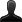CrashBot
• Owned by
Not owned by anyone
• Estimated time
Not estimated
• Category
Not determined
• Resolution
Not determined
• Priority
Not determined
• Reproducability
Not determined
• Severity
Not determined
• Targetted for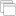Not determined
• OS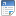Linux
• Architectureamd64
• Fixed inNot determined
Issue description
Illegal storage access

``` Instruction address: 0x617895
Memory accessed: 0x0
Stack pointer: 0x7ffdf2a22010
```

Callstack:
``` arx!0x5b7edf
arx!0x617895
arx!0x512136
arx!0x43e23f
arx!0x648802
arx!0x47158b
arx!0x47a1a1
arx!0x691dba
arx!0x6972b8
arx!0x698578
arx!0x4393e6
libc-2.31.so!0x7fc8f591e0b3
arx!0x43a7dd
```

GDB stack trace:
``` [New LWP 2496]
[New LWP 2497]
[New LWP 2498]
[New LWP 2499]
[New LWP 2500]
[New LWP 2501]
[New LWP 2502]
[New LWP 2503]
[New LWP 2504]
[New LWP 2505]
[New LWP 2509]
[New LWP 2511]
[New LWP 2512]
[New LWP 2513]
[New LWP 2514]
[New LWP 20271]
0x00007fc8f59d73bf in __GI___clock_nanosleep (clock_id=clock_id@entry=0, flags=flags@entry=0, req=0x7ffdf2a21a30, rem=0x0) at ../sysdeps/unix/sysv/linux/clock_nanosleep.c:78
Id   Target Id                                       Frame
* 1    Thread 0x7fc8f58f4740 (LWP 2487) "arx"          0x00007fc8f59d73bf in __GI___clock_nanosleep (clock_id=clock_id@entry=0, flags=flags@entry=0, req=0x7ffdf2a21a30, rem=0x0) at ../sysdeps/unix/sysv/linux/clock_nanosleep.c:78
2    Thread 0x7fc8ed041700 (LWP 2496) "arx:cs0"      futex_wait_cancelable (private=<optimized out>, expected=0, futex_word=0x201b258) at ../sysdeps/nptl/futex-internal.h:183
3    Thread 0x7fc8e7fff700 (LWP 2497) "arx:disk\$0"   futex_wait_cancelable (private=<optimized out>, expected=0, futex_word=0x201e38c) at ../sysdeps/nptl/futex-internal.h:183
4    Thread 0x7fc8e77fe700 (LWP 2498) "arx:disk\$1"   futex_wait_cancelable (private=<optimized out>, expected=0, futex_word=0x201e388) at ../sysdeps/nptl/futex-internal.h:183
5    Thread 0x7fc8e6ffd700 (LWP 2499) "arx:disk\$2"   futex_wait_cancelable (private=<optimized out>, expected=0, futex_word=0x201e388) at ../sysdeps/nptl/futex-internal.h:183
6    Thread 0x7fc8e67fc700 (LWP 2500) "arx:disk\$3"   futex_wait_cancelable (private=<optimized out>, expected=0, futex_word=0x201e388) at ../sysdeps/nptl/futex-internal.h:183
7    Thread 0x7fc8e5ffb700 (LWP 2501) "arx:sh0"      futex_wait_cancelable (private=<optimized out>, expected=0, futex_word=0x201d7d8) at ../sysdeps/nptl/futex-internal.h:183
8    Thread 0x7fc8e57fa700 (LWP 2502) "arx:sh1"      futex_wait_cancelable (private=<optimized out>, expected=0, futex_word=0x201d7dc) at ../sysdeps/nptl/futex-internal.h:183
9    Thread 0x7fc8e4ff9700 (LWP 2503) "arx:sh2"      futex_wait_cancelable (private=<optimized out>, expected=0, futex_word=0x201d7dc) at ../sysdeps/nptl/futex-internal.h:183
10   Thread 0x7fc8d7fff700 (LWP 2504) "arx:shlo0"    futex_wait_cancelable (private=<optimized out>, expected=0, futex_word=0x201dee0) at ../sysdeps/nptl/futex-internal.h:183
11   Thread 0x7fc8d77fe700 (LWP 2505) "arx:shlo1"    futex_wait_cancelable (private=<optimized out>, expected=0, futex_word=0x201dee0) at ../sysdeps/nptl/futex-internal.h:183
12   Thread 0x7fc8d6ffd700 (LWP 2509) "arx:gdrv0"    futex_wait_cancelable (private=<optimized out>, expected=0, futex_word=0x21e1314) at ../sysdeps/nptl/futex-internal.h:183
13   Thread 0x7fc8d64c3700 (LWP 2511) "threaded-ml"  0x00007fc8f5a0caff in __GI___poll (fds=0x7fc8ac009cb0, nfds=3, timeout=1020) at ../sysdeps/unix/sysv/linux/poll.c:29
14   Thread 0x7fc8d5cc2700 (LWP 2512) "arx"          futex_wait_cancelable (private=<optimized out>, expected=0, futex_word=0x264240c) at ../sysdeps/nptl/futex-internal.h:183
15   Thread 0x7fc8d529a700 (LWP 2513) "arx"          futex_abstimed_wait_cancelable (private=0, abstime=0x0, clockid=0, expected=0, futex_word=0x1ff2e00) at ../sysdeps/nptl/futex-internal.h:320
16   Thread 0x7fc8d5099700 (LWP 2514) "Sound Update" 0x00007fc8f59d73bf in __GI___clock_nanosleep (clock_id=clock_id@entry=0, flags=flags@entry=0, req=0x7fc8d5098e70, rem=0x0) at ../sysdeps/unix/sysv/linux/clock_nanosleep.c:78
17   Thread 0x7fc896d5e700 (LWP 20271) "Pathfinder"  0x00007fc8f59d73bf in __GI___clock_nanosleep (clock_id=clock_id@entry=0, flags=flags@entry=0, req=0x7fc896d5de80, rem=0x0) at ../sysdeps/unix/sysv/linux/clock_nanosleep.c:78

#0  0x00007fc8f59d73bf in __GI___clock_nanosleep (clock_id=clock_id@entry=0, flags=flags@entry=0, req=0x7fc896d5de80, rem=0x0) at ../sysdeps/unix/sysv/linux/clock_nanosleep.c:78
sc_cancel_oldtype = 0
sc_ret = <optimized out>
r = <optimized out>
#1  0x00007fc8f59dd047 in __GI___nanosleep (requested_time=<optimized out>, remaining=<optimized out>) at nanosleep.c:27
ret = <optimized out>
#2  0x00000000006c1f05 in ?? ()
No symbol table info available.
#3  0x00000000005a5959 in ?? ()
No symbol table info available.
ret = <optimized out>
pd = <optimized out>
unwind_buf = {cancel_jmp_buf = {{jmp_buf = {140499500787456, -1135049281572039933, 140728674151358, 140728674151359, 140728674151680, 140499500785600, 1129931930055611139, 1130004882941809411}, mask_was_saved = 0}}, priv = {pad = {0x0, 0x0, 0x0, 0x0}, data = {prev = 0x0, cleanup = 0x0, canceltype = 0}}}
not_first_call = 0
#5  0x00007fc8f5a19293 in clone () at ../sysdeps/unix/sysv/linux/x86_64/clone.S:95
No locals.

#0  0x00007fc8f59d73bf in __GI___clock_nanosleep (clock_id=clock_id@entry=0, flags=flags@entry=0, req=0x7fc8d5098e70, rem=0x0) at ../sysdeps/unix/sysv/linux/clock_nanosleep.c:78
sc_cancel_oldtype = 0
sc_ret = <optimized out>
r = <optimized out>
#1  0x00007fc8f59dd047 in __GI___nanosleep (requested_time=<optimized out>, remaining=<optimized out>) at nanosleep.c:27
ret = <optimized out>
#2  0x00000000006b2b00 in ?? ()
No symbol table info available.
#3  0x00000000005a5959 in ?? ()
No symbol table info available.
ret = <optimized out>
pd = <optimized out>
unwind_buf = {cancel_jmp_buf = {{jmp_buf = {140500544362240, -1135049281572039933, 140728674165182, 140728674165183, 140728674165456, 140500544360384, 1130074349560533763, 1130004882941809411}, mask_was_saved = 0}}, priv = {pad = {0x0, 0x0, 0x0, 0x0}, data = {prev = 0x0, cleanup = 0x0, canceltype = 0}}}
not_first_call = 0
#5  0x00007fc8f5a19293 in clone () at ../sysdeps/unix/sysv/linux/x86_64/clone.S:95
No locals.

#0  futex_abstimed_wait_cancelable (private=0, abstime=0x0, clockid=0, expected=0, futex_word=0x1ff2e00) at ../sysdeps/nptl/futex-internal.h:320
__ret = -512
op = 393
__ret = <optimized out>
oldtype = 0
err = <optimized out>
oldtype = <optimized out>
err = <optimized out>
__ret = <optimized out>
clockbit = <optimized out>
op = <optimized out>
__ret = <optimized out>
resultvar = <optimized out>
__arg6 = <optimized out>
__arg5 = <optimized out>
__arg4 = <optimized out>
__arg3 = <optimized out>
__arg2 = <optimized out>
__arg1 = <optimized out>
_a6 = <optimized out>
_a5 = <optimized out>
_a4 = <optimized out>
_a3 = <optimized out>
_a2 = <optimized out>
_a1 = <optimized out>
#1  do_futex_wait (sem=sem@entry=0x1ff2e00, abstime=0x0, clockid=0) at sem_waitcommon.c:112
err = <optimized out>
#2  0x00007fc8f5e32548 in __new_sem_wait_slow (sem=0x1ff2e00, abstime=0x0, clockid=0) at sem_waitcommon.c:184
_buffer = {__routine = 0x7fc8f5e32400 <__sem_wait_cleanup>, __arg = 0x1ff2e00, __canceltype = 0, __prev = 0x0}
err = <optimized out>
d = 0
#3  0x00007fc8f5d7f445 in ?? () from /media/cammera/Datos/Juegos/Arx Fatalis/arx-libertatis-1.2-linux/bin/amd64/libopenal.so.1
No symbol table info available.
#4  0x00007fc8f5d50117 in ?? () from /media/cammera/Datos/Juegos/Arx Fatalis/arx-libertatis-1.2-linux/bin/amd64/libopenal.so.1
No symbol table info available.
ret = <optimized out>
pd = <optimized out>
unwind_buf = {cancel_jmp_buf = {{jmp_buf = {140500546463488, -1135049281572039933, 140728674163854, 140728674163855, 140728674164000, 140500546461632, 1130074623901569795, 1130004882941809411}, mask_was_saved = 0}}, priv = {pad = {0x0, 0x0, 0x0, 0x0}, data = {prev = 0x0, cleanup = 0x0, canceltype = 0}}}
not_first_call = 0
#6  0x00007fc8f5a19293 in clone () at ../sysdeps/unix/sysv/linux/x86_64/clone.S:95
No locals.

#0  futex_wait_cancelable (private=<optimized out>, expected=0, futex_word=0x264240c) at ../sysdeps/nptl/futex-internal.h:183
__ret = -512
oldtype = 0
err = <optimized out>
oldtype = <optimized out>
err = <optimized out>
__ret = <optimized out>
resultvar = <optimized out>
__arg4 = <optimized out>
__arg3 = <optimized out>
__arg2 = <optimized out>
__arg1 = <optimized out>
_a4 = <optimized out>
_a3 = <optimized out>
_a2 = <optimized out>
_a1 = <optimized out>
spin = 0
buffer = {__routine = 0x7fc8f5e2f050 <__condvar_cleanup_waiting>, __arg = 0x7fc8d5cc1e20, __canceltype = 8192, __prev = 0x0}
cbuffer = {wseq = 1972375, cond = 0x26423e0, mutex = 0x26449c0, private = 0}
err = <optimized out>
g = 1
flags = <optimized out>
g1_start = <optimized out>
signals = <optimized out>
result = 0
wseq = 1972375
seq = 986187
private = <optimized out>
maxspin = <optimized out>
err = <optimized out>
result = <optimized out>
wseq = <optimized out>
g = <optimized out>
seq = <optimized out>
flags = <optimized out>
private = <optimized out>
signals = <optimized out>
g1_start = <optimized out>
spin = <optimized out>
buffer = <optimized out>
cbuffer = <optimized out>
s = <optimized out>
No locals.
#3  0x00007fc8ec07287c in pa_threaded_mainloop_wait () from /lib/x86_64-linux-gnu/libpulse.so.0
No symbol table info available.
#4  0x00007fc8f5d53da6 in ?? () from /media/cammera/Datos/Juegos/Arx Fatalis/arx-libertatis-1.2-linux/bin/amd64/libopenal.so.1
No symbol table info available.
#5  0x00007fc8f5d50117 in ?? () from /media/cammera/Datos/Juegos/Arx Fatalis/arx-libertatis-1.2-linux/bin/amd64/libopenal.so.1
No symbol table info available.
ret = <optimized out>
pd = <optimized out>
unwind_buf = {cancel_jmp_buf = {{jmp_buf = {140500557113088, -1135049281572039933, 140728674163694, 140728674163695, 140728674163840, 140500557111232, 1130074928844247811, 1130004882941809411}, mask_was_saved = 0}}, priv = {pad = {0x0, 0x0, 0x0, 0x0}, data = {prev = 0x0, cleanup = 0x0, canceltype = 0}}}
not_first_call = 0
#7  0x00007fc8f5a19293 in clone () at ../sysdeps/unix/sysv/linux/x86_64/clone.S:95
No locals.

#0  0x00007fc8f5a0caff in __GI___poll (fds=0x7fc8ac009cb0, nfds=3, timeout=1020) at ../sysdeps/unix/sysv/linux/poll.c:29
resultvar = 18446744073709551100
sc_cancel_oldtype = 0
sc_ret = <optimized out>
#1  0x00007fc8ec0721d6 in ?? () from /lib/x86_64-linux-gnu/libpulse.so.0
No symbol table info available.
#2  0x00007fc8ec063841 in pa_mainloop_poll () from /lib/x86_64-linux-gnu/libpulse.so.0
No symbol table info available.
#3  0x00007fc8ec063ec3 in pa_mainloop_iterate () from /lib/x86_64-linux-gnu/libpulse.so.0
No symbol table info available.
#4  0x00007fc8ec063f70 in pa_mainloop_run () from /lib/x86_64-linux-gnu/libpulse.so.0
No symbol table info available.
#5  0x00007fc8ec07211d in ?? () from /lib/x86_64-linux-gnu/libpulse.so.0
No symbol table info available.
#6  0x00007fc8e40ea72c in ?? () from /usr/lib/x86_64-linux-gnu/pulseaudio/libpulsecommon-13.99.so
No symbol table info available.
ret = <optimized out>
pd = <optimized out>
unwind_buf = {cancel_jmp_buf = {{jmp_buf = {140500565505792, -1135049281572039933, 140728674163806, 140728674163807, 140728674163808, 140500565503936, 1130071631919977219, 1130004882941809411}, mask_was_saved = 0}}, priv = {pad = {0x0, 0x0, 0x0, 0x0}, data = {prev = 0x0, cleanup = 0x0, canceltype = 0}}}
not_first_call = 0
#8  0x00007fc8f5a19293 in clone () at ../sysdeps/unix/sysv/linux/x86_64/clone.S:95
No locals.

#0  futex_wait_cancelable (private=<optimized out>, expected=0, futex_word=0x21e1314) at ../sysdeps/nptl/futex-internal.h:183
__ret = -512
oldtype = 0
err = <optimized out>
oldtype = <optimized out>
err = <optimized out>
__ret = <optimized out>
resultvar = <optimized out>
__arg4 = <optimized out>
__arg3 = <optimized out>
__arg2 = <optimized out>
__arg1 = <optimized out>
_a4 = <optimized out>
_a3 = <optimized out>
_a2 = <optimized out>
_a1 = <optimized out>
spin = 0
buffer = {__routine = 0x7fc8f5e2f050 <__condvar_cleanup_waiting>, __arg = 0x7fc8d6ffccf0, __canceltype = 0, __prev = 0x0}
cbuffer = {wseq = 1626959, cond = 0x21e12e8, mutex = 0x21e12c0, private = 0}
err = <optimized out>
g = 1
flags = <optimized out>
g1_start = <optimized out>
signals = <optimized out>
result = 0
wseq = 1626959
seq = 813479
private = <optimized out>
maxspin = <optimized out>
err = <optimized out>
result = <optimized out>
wseq = <optimized out>
g = <optimized out>
seq = <optimized out>
flags = <optimized out>
private = <optimized out>
signals = <optimized out>
g1_start = <optimized out>
spin = <optimized out>
buffer = <optimized out>
cbuffer = <optimized out>
s = <optimized out>
No locals.
#3  0x00007fc8f2d1ee7b in ?? () from /usr/lib/x86_64-linux-gnu/dri/radeonsi_dri.so
No symbol table info available.
#4  0x00007fc8f2d1ea7b in ?? () from /usr/lib/x86_64-linux-gnu/dri/radeonsi_dri.so
No symbol table info available.
ret = <optimized out>
pd = <optimized out>
unwind_buf = {cancel_jmp_buf = {{jmp_buf = {140500577277696, -1135049281572039933, 140728674161454, 140728674161455, 140728674161456, 140500577275840, 1130072891419136771, 1130004882941809411}, mask_was_saved = 0}}, priv = {pad = {0x0, 0x0, 0x0, 0x0}, data = {prev = 0x0, cleanup = 0x0, canceltype = 0}}}
not_first_call = 0
#6  0x00007fc8f5a19293 in clone () at ../sysdeps/unix/sysv/linux/x86_64/clone.S:95
No locals.

#0  futex_wait_cancelable (private=<optimized out>, expected=0, futex_word=0x201dee0) at ../sysdeps/nptl/futex-internal.h:183
__ret = -512
oldtype = 0
err = <optimized out>
oldtype = <optimized out>
err = <optimized out>
__ret = <optimized out>
resultvar = <optimized out>
__arg4 = <optimized out>
__arg3 = <optimized out>
__arg2 = <optimized out>
__arg1 = <optimized out>
_a4 = <optimized out>
_a3 = <optimized out>
_a2 = <optimized out>
_a1 = <optimized out>
spin = 0
buffer = {__routine = 0x7fc8f5e2f050 <__condvar_cleanup_waiting>, __arg = 0x7fc8d77fdcf0, __canceltype = 90738384, __prev = 0x0}
cbuffer = {wseq = 8, cond = 0x201deb8, mutex = 0x201de90, private = 0}
err = <optimized out>
g = 0
flags = <optimized out>
g1_start = <optimized out>
signals = <optimized out>
result = 0
wseq = 8
seq = 4
private = <optimized out>
maxspin = <optimized out>
err = <optimized out>
result = <optimized out>
wseq = <optimized out>
g = <optimized out>
seq = <optimized out>
flags = <optimized out>
private = <optimized out>
signals = <optimized out>
g1_start = <optimized out>
spin = <optimized out>
buffer = <optimized out>
cbuffer = <optimized out>
s = <optimized out>
No locals.
#3  0x00007fc8f2d1ee7b in ?? () from /usr/lib/x86_64-linux-gnu/dri/radeonsi_dri.so
No symbol table info available.
#4  0x00007fc8f2d1ea7b in ?? () from /usr/lib/x86_64-linux-gnu/dri/radeonsi_dri.so
No symbol table info available.
ret = <optimized out>
pd = <optimized out>
unwind_buf = {cancel_jmp_buf = {{jmp_buf = {140500585670400, -1135049281572039933, 140728674159982, 140728674159983, 140728674159984, 140500585668544, 1130069592347382531, 1130004882941809411}, mask_was_saved = 0}}, priv = {pad = {0x0, 0x0, 0x0, 0x0}, data = {prev = 0x0, cleanup = 0x0, canceltype = 0}}}
not_first_call = 0
#6  0x00007fc8f5a19293 in clone () at ../sysdeps/unix/sysv/linux/x86_64/clone.S:95
No locals.

#0  futex_wait_cancelable (private=<optimized out>, expected=0, futex_word=0x201dee0) at ../sysdeps/nptl/futex-internal.h:183
__ret = -512
oldtype = 0
err = <optimized out>
oldtype = <optimized out>
err = <optimized out>
__ret = <optimized out>
resultvar = <optimized out>
__arg4 = <optimized out>
__arg3 = <optimized out>
__arg2 = <optimized out>
__arg1 = <optimized out>
_a4 = <optimized out>
_a3 = <optimized out>
_a2 = <optimized out>
_a1 = <optimized out>
spin = 0
buffer = {__routine = 0x7fc8f5e2f050 <__condvar_cleanup_waiting>, __arg = 0x7fc8d7ffecf0, __canceltype = 33673088, __prev = 0x0}
cbuffer = {wseq = 6, cond = 0x201deb8, mutex = 0x201de90, private = 0}
err = <optimized out>
g = 0
flags = <optimized out>
g1_start = <optimized out>
signals = <optimized out>
result = 0
wseq = 6
seq = 3
private = <optimized out>
maxspin = <optimized out>
err = <optimized out>
result = <optimized out>
wseq = <optimized out>
g = <optimized out>
seq = <optimized out>
flags = <optimized out>
private = <optimized out>
signals = <optimized out>
g1_start = <optimized out>
spin = <optimized out>
buffer = <optimized out>
cbuffer = <optimized out>
s = <optimized out>
No locals.
#3  0x00007fc8f2d1ee7b in ?? () from /usr/lib/x86_64-linux-gnu/dri/radeonsi_dri.so
No symbol table info available.
#4  0x00007fc8f2d1ea7b in ?? () from /usr/lib/x86_64-linux-gnu/dri/radeonsi_dri.so
No symbol table info available.
ret = <optimized out>
pd = <optimized out>
unwind_buf = {cancel_jmp_buf = {{jmp_buf = {140500594063104, -1135049281572039933, 140728674159982, 140728674159983, 140728674159984, 140500594061248, 1130070693469623043, 1130004882941809411}, mask_was_saved = 0}}, priv = {pad = {0x0, 0x0, 0x0, 0x0}, data = {prev = 0x0, cleanup = 0x0, canceltype = 0}}}
not_first_call = 0
#6  0x00007fc8f5a19293 in clone () at ../sysdeps/unix/sysv/linux/x86_64/clone.S:95
No locals.

#0  futex_wait_cancelable (private=<optimized out>, expected=0, futex_word=0x201d7dc) at ../sysdeps/nptl/futex-internal.h:183
__ret = -512
oldtype = 0
err = <optimized out>
oldtype = <optimized out>
err = <optimized out>
__ret = <optimized out>
resultvar = <optimized out>
__arg4 = <optimized out>
__arg3 = <optimized out>
__arg2 = <optimized out>
__arg1 = <optimized out>
_a4 = <optimized out>
_a3 = <optimized out>
_a2 = <optimized out>
_a1 = <optimized out>
spin = 0
buffer = {__routine = 0x7fc8f5e2f050 <__condvar_cleanup_waiting>, __arg = 0x7fc8e4ff8cf0, __canceltype = 0, __prev = 0x0}
cbuffer = {wseq = 65, cond = 0x201d7b0, mutex = 0x201d788, private = 0}
err = <optimized out>
g = 1
flags = <optimized out>
g1_start = <optimized out>
signals = <optimized out>
result = 0
wseq = 65
seq = 32
private = <optimized out>
maxspin = <optimized out>
err = <optimized out>
result = <optimized out>
wseq = <optimized out>
g = <optimized out>
seq = <optimized out>
flags = <optimized out>
private = <optimized out>
signals = <optimized out>
g1_start = <optimized out>
spin = <optimized out>
buffer = <optimized out>
cbuffer = <optimized out>
s = <optimized out>
No locals.
#3  0x00007fc8f2d1ee7b in ?? () from /usr/lib/x86_64-linux-gnu/dri/radeonsi_dri.so
No symbol table info available.
#4  0x00007fc8f2d1ea7b in ?? () from /usr/lib/x86_64-linux-gnu/dri/radeonsi_dri.so
No symbol table info available.
ret = <optimized out>
pd = <optimized out>
unwind_buf = {cancel_jmp_buf = {{jmp_buf = {140500812142336, -1135049281572039933, 140728674159982, 140728674159983, 140728674159984, 140500812140480, 1130042102946075395, 1130004882941809411}, mask_was_saved = 0}}, priv = {pad = {0x0, 0x0, 0x0, 0x0}, data = {prev = 0x0, cleanup = 0x0, canceltype = 0}}}
not_first_call = 0
#6  0x00007fc8f5a19293 in clone () at ../sysdeps/unix/sysv/linux/x86_64/clone.S:95
No locals.

#0  futex_wait_cancelable (private=<optimized out>, expected=0, futex_word=0x201d7dc) at ../sysdeps/nptl/futex-internal.h:183
__ret = -512
oldtype = 0
err = <optimized out>
oldtype = <optimized out>
err = <optimized out>
__ret = <optimized out>
resultvar = <optimized out>
__arg4 = <optimized out>
__arg3 = <optimized out>
__arg2 = <optimized out>
__arg1 = <optimized out>
_a4 = <optimized out>
_a3 = <optimized out>
_a2 = <optimized out>
_a1 = <optimized out>
spin = 0
buffer = {__routine = 0x7fc8f5e2f050 <__condvar_cleanup_waiting>, __arg = 0x7fc8e57f9cf0, __canceltype = 0, __prev = 0x0}
cbuffer = {wseq = 67, cond = 0x201d7b0, mutex = 0x201d788, private = 0}
err = <optimized out>
g = 1
flags = <optimized out>
g1_start = <optimized out>
signals = <optimized out>
result = 0
wseq = 67
seq = 33
private = <optimized out>
maxspin = <optimized out>
err = <optimized out>
result = <optimized out>
wseq = <optimized out>
g = <optimized out>
seq = <optimized out>
flags = <optimized out>
private = <optimized out>
signals = <optimized out>
g1_start = <optimized out>
spin = <optimized out>
buffer = <optimized out>
cbuffer = <optimized out>
s = <optimized out>
No locals.
#3  0x00007fc8f2d1ee7b in ?? () from /usr/lib/x86_64-linux-gnu/dri/radeonsi_dri.so
No symbol table info available.
#4  0x00007fc8f2d1ea7b in ?? () from /usr/lib/x86_64-linux-gnu/dri/radeonsi_dri.so
No symbol table info available.
ret = <optimized out>
pd = <optimized out>
unwind_buf = {cancel_jmp_buf = {{jmp_buf = {140500820535040, -1135049281572039933, 140728674159982, 140728674159983, 140728674159984, 140500820533184, 1130038803874321155, 1130004882941809411}, mask_was_saved = 0}}, priv = {pad = {0x0, 0x0, 0x0, 0x0}, data = {prev = 0x0, cleanup = 0x0, canceltype = 0}}}
not_first_call = 0
#6  0x00007fc8f5a19293 in clone () at ../sysdeps/unix/sysv/linux/x86_64/clone.S:95
No locals.

#0  futex_wait_cancelable (private=<optimized out>, expected=0, futex_word=0x201d7d8) at ../sysdeps/nptl/futex-internal.h:183
__ret = -512
oldtype = 0
err = <optimized out>
oldtype = <optimized out>
err = <optimized out>
__ret = <optimized out>
resultvar = <optimized out>
__arg4 = <optimized out>
__arg3 = <optimized out>
__arg2 = <optimized out>
__arg1 = <optimized out>
_a4 = <optimized out>
_a3 = <optimized out>
_a2 = <optimized out>
_a1 = <optimized out>
spin = 0
buffer = {__routine = 0x7fc8f5e2f050 <__condvar_cleanup_waiting>, __arg = 0x7fc8e5ffacf0, __canceltype = 0, __prev = 0x0}
cbuffer = {wseq = 68, cond = 0x201d7b0, mutex = 0x201d788, private = 0}
err = <optimized out>
g = 0
flags = <optimized out>
g1_start = <optimized out>
signals = <optimized out>
result = 0
wseq = 68
seq = 34
private = <optimized out>
maxspin = <optimized out>
err = <optimized out>
result = <optimized out>
wseq = <optimized out>
g = <optimized out>
seq = <optimized out>
flags = <optimized out>
private = <optimized out>
signals = <optimized out>
g1_start = <optimized out>
spin = <optimized out>
buffer = <optimized out>
cbuffer = <optimized out>
s = <optimized out>
No locals.
#3  0x00007fc8f2d1ee7b in ?? () from /usr/lib/x86_64-linux-gnu/dri/radeonsi_dri.so
No symbol table info available.
#4  0x00007fc8f2d1ea7b in ?? () from /usr/lib/x86_64-linux-gnu/dri/radeonsi_dri.so
No symbol table info available.
ret = <optimized out>
pd = <optimized out>
unwind_buf = {cancel_jmp_buf = {{jmp_buf = {140500828927744, -1135049281572039933, 140728674159982, 140728674159983, 140728674159984, 140500828925888, 1130039904996561667, 1130004882941809411}, mask_was_saved = 0}}, priv = {pad = {0x0, 0x0, 0x0, 0x0}, data = {prev = 0x0, cleanup = 0x0, canceltype = 0}}}
not_first_call = 0
#6  0x00007fc8f5a19293 in clone () at ../sysdeps/unix/sysv/linux/x86_64/clone.S:95
No locals.

#0  futex_wait_cancelable (private=<optimized out>, expected=0, futex_word=0x201e388) at ../sysdeps/nptl/futex-internal.h:183
__ret = -512
oldtype = 0
err = <optimized out>
oldtype = <optimized out>
err = <optimized out>
__ret = <optimized out>
resultvar = <optimized out>
__arg4 = <optimized out>
__arg3 = <optimized out>
__arg2 = <optimized out>
__arg1 = <optimized out>
_a4 = <optimized out>
_a3 = <optimized out>
_a2 = <optimized out>
_a1 = <optimized out>
spin = 0
buffer = {__routine = 0x7fc8f5e2f050 <__condvar_cleanup_waiting>, __arg = 0x7fc8e67fbcf0, __canceltype = 0, __prev = 0x0}
cbuffer = {wseq = 6, cond = 0x201e360, mutex = 0x201e338, private = 0}
err = <optimized out>
g = 0
flags = <optimized out>
g1_start = <optimized out>
signals = <optimized out>
result = 0
wseq = 6
seq = 3
private = <optimized out>
maxspin = <optimized out>
err = <optimized out>
result = <optimized out>
wseq = <optimized out>
g = <optimized out>
seq = <optimized out>
flags = <optimized out>
private = <optimized out>
signals = <optimized out>
g1_start = <optimized out>
spin = <optimized out>
buffer = <optimized out>
cbuffer = <optimized out>
s = <optimized out>
No locals.
#3  0x00007fc8f2d1ee7b in ?? () from /usr/lib/x86_64-linux-gnu/dri/radeonsi_dri.so
No symbol table info available.
#4  0x00007fc8f2d1ea7b in ?? () from /usr/lib/x86_64-linux-gnu/dri/radeonsi_dri.so
No symbol table info available.
ret = <optimized out>
pd = <optimized out>
unwind_buf = {cancel_jmp_buf = {{jmp_buf = {140500837320448, -1135049281572039933, 140728674159454, 140728674159455, 140728674159456, 140500837318592, 1130036605924807427, 1130004882941809411}, mask_was_saved = 0}}, priv = {pad = {0x0, 0x0, 0x0, 0x0}, data = {prev = 0x0, cleanup = 0x0, canceltype = 0}}}
not_first_call = 0
#6  0x00007fc8f5a19293 in clone () at ../sysdeps/unix/sysv/linux/x86_64/clone.S:95
No locals.

#0  futex_wait_cancelable (private=<optimized out>, expected=0, futex_word=0x201e388) at ../sysdeps/nptl/futex-internal.h:183
__ret = -512
oldtype = 0
err = <optimized out>
oldtype = <optimized out>
err = <optimized out>
__ret = <optimized out>
resultvar = <optimized out>
__arg4 = <optimized out>
__arg3 = <optimized out>
__arg2 = <optimized out>
__arg1 = <optimized out>
_a4 = <optimized out>
_a3 = <optimized out>
_a2 = <optimized out>
_a1 = <optimized out>
spin = 0
buffer = {__routine = 0x7fc8f5e2f050 <__condvar_cleanup_waiting>, __arg = 0x7fc8e6ffccf0, __canceltype = 0, __prev = 0x0}
cbuffer = {wseq = 2, cond = 0x201e360, mutex = 0x201e338, private = 0}
err = <optimized out>
g = 0
flags = <optimized out>
g1_start = <optimized out>
signals = <optimized out>
result = 0
wseq = 2
seq = 1
private = <optimized out>
maxspin = <optimized out>
err = <optimized out>
result = <optimized out>
wseq = <optimized out>
g = <optimized out>
seq = <optimized out>
flags = <optimized out>
private = <optimized out>
signals = <optimized out>
g1_start = <optimized out>
spin = <optimized out>
buffer = <optimized out>
cbuffer = <optimized out>
s = <optimized out>
No locals.
#3  0x00007fc8f2d1ee7b in ?? () from /usr/lib/x86_64-linux-gnu/dri/radeonsi_dri.so
No symbol table info available.
#4  0x00007fc8f2d1ea7b in ?? () from /usr/lib/x86_64-linux-gnu/dri/radeonsi_dri.so
No symbol table info available.
ret = <optimized out>
pd = <optimized out>
unwind_buf = {cancel_jmp_buf = {{jmp_buf = {140500845713152, -1135049281572039933, 140728674159454, 140728674159455, 140728674159456, 140500845711296, 1130037707047047939, 1130004882941809411}, mask_was_saved = 0}}, priv = {pad = {0x0, 0x0, 0x0, 0x0}, data = {prev = 0x0, cleanup = 0x0, canceltype = 0}}}
not_first_call = 0
#6  0x00007fc8f5a19293 in clone () at ../sysdeps/unix/sysv/linux/x86_64/clone.S:95
No locals.

#0  futex_wait_cancelable (private=<optimized out>, expected=0, futex_word=0x201e388) at ../sysdeps/nptl/futex-internal.h:183
__ret = -512
oldtype = 0
err = <optimized out>
oldtype = <optimized out>
err = <optimized out>
__ret = <optimized out>
resultvar = <optimized out>
__arg4 = <optimized out>
__arg3 = <optimized out>
__arg2 = <optimized out>
__arg1 = <optimized out>
_a4 = <optimized out>
_a3 = <optimized out>
_a2 = <optimized out>
_a1 = <optimized out>
spin = 0
buffer = {__routine = 0x7fc8f5e2f050 <__condvar_cleanup_waiting>, __arg = 0x7fc8e77fdcf0, __canceltype = 0, __prev = 0x0}
cbuffer = {wseq = 4, cond = 0x201e360, mutex = 0x201e338, private = 0}
err = <optimized out>
g = 0
flags = <optimized out>
g1_start = <optimized out>
signals = <optimized out>
result = 0
wseq = 4
seq = 2
private = <optimized out>
maxspin = <optimized out>
err = <optimized out>
result = <optimized out>
wseq = <optimized out>
g = <optimized out>
seq = <optimized out>
flags = <optimized out>
private = <optimized out>
signals = <optimized out>
g1_start = <optimized out>
spin = <optimized out>
buffer = <optimized out>
cbuffer = <optimized out>
s = <optimized out>
No locals.
#3  0x00007fc8f2d1ee7b in ?? () from /usr/lib/x86_64-linux-gnu/dri/radeonsi_dri.so
No symbol table info available.
#4  0x00007fc8f2d1ea7b in ?? () from /usr/lib/x86_64-linux-gnu/dri/radeonsi_dri.so
No symbol table info available.
ret = <optimized out>
pd = <optimized out>
unwind_buf = {cancel_jmp_buf = {{jmp_buf = {140500854105856, -1135049281572039933, 140728674159454, 140728674159455, 140728674159456, 140500854104000, 1130034407975293699, 1130004882941809411}, mask_was_saved = 0}}, priv = {pad = {0x0, 0x0, 0x0, 0x0}, data = {prev = 0x0, cleanup = 0x0, canceltype = 0}}}
not_first_call = 0
#6  0x00007fc8f5a19293 in clone () at ../sysdeps/unix/sysv/linux/x86_64/clone.S:95
No locals.

#0  futex_wait_cancelable (private=<optimized out>, expected=0, futex_word=0x201e38c) at ../sysdeps/nptl/futex-internal.h:183
__ret = -512
oldtype = 0
err = <optimized out>
oldtype = <optimized out>
err = <optimized out>
__ret = <optimized out>
resultvar = <optimized out>
__arg4 = <optimized out>
__arg3 = <optimized out>
__arg2 = <optimized out>
__arg1 = <optimized out>
_a4 = <optimized out>
_a3 = <optimized out>
_a2 = <optimized out>
_a1 = <optimized out>
spin = 0
buffer = {__routine = 0x7fc8f5e2f050 <__condvar_cleanup_waiting>, __arg = 0x7fc8e7ffecf0, __canceltype = 892482355, __prev = 0x0}
cbuffer = {wseq = 9, cond = 0x201e360, mutex = 0x201e338, private = 0}
err = <optimized out>
g = 1
flags = <optimized out>
g1_start = <optimized out>
signals = <optimized out>
result = 0
wseq = 9
seq = 4
private = <optimized out>
maxspin = <optimized out>
err = <optimized out>
result = <optimized out>
wseq = <optimized out>
g = <optimized out>
seq = <optimized out>
flags = <optimized out>
private = <optimized out>
signals = <optimized out>
g1_start = <optimized out>
spin = <optimized out>
buffer = <optimized out>
cbuffer = <optimized out>
s = <optimized out>
No locals.
#3  0x00007fc8f2d1ee7b in ?? () from /usr/lib/x86_64-linux-gnu/dri/radeonsi_dri.so
No symbol table info available.
#4  0x00007fc8f2d1ea7b in ?? () from /usr/lib/x86_64-linux-gnu/dri/radeonsi_dri.so
No symbol table info available.
ret = <optimized out>
pd = <optimized out>
unwind_buf = {cancel_jmp_buf = {{jmp_buf = {140500862498560, -1135049281572039933, 140728674159454, 140728674159455, 140728674159456, 140500862496704, 1130035509097534211, 1130004882941809411}, mask_was_saved = 0}}, priv = {pad = {0x0, 0x0, 0x0, 0x0}, data = {prev = 0x0, cleanup = 0x0, canceltype = 0}}}
not_first_call = 0
#6  0x00007fc8f5a19293 in clone () at ../sysdeps/unix/sysv/linux/x86_64/clone.S:95
No locals.

#0  futex_wait_cancelable (private=<optimized out>, expected=0, futex_word=0x201b258) at ../sysdeps/nptl/futex-internal.h:183
__ret = -512
oldtype = 0
err = <optimized out>
oldtype = <optimized out>
err = <optimized out>
__ret = <optimized out>
resultvar = <optimized out>
__arg4 = <optimized out>
__arg3 = <optimized out>
__arg2 = <optimized out>
__arg1 = <optimized out>
_a4 = <optimized out>
_a3 = <optimized out>
_a2 = <optimized out>
_a1 = <optimized out>
spin = 0
buffer = {__routine = 0x7fc8f5e2f050 <__condvar_cleanup_waiting>, __arg = 0x7fc8ed040cf0, __canceltype = 49, __prev = 0x0}
cbuffer = {wseq = 2226896, cond = 0x201b230, mutex = 0x201b208, private = 0}
err = <optimized out>
g = 0
flags = <optimized out>
g1_start = <optimized out>
signals = <optimized out>
result = 0
wseq = 2226896
seq = 1113448
private = <optimized out>
maxspin = <optimized out>
err = <optimized out>
result = <optimized out>
wseq = <optimized out>
g = <optimized out>
seq = <optimized out>
flags = <optimized out>
private = <optimized out>
signals = <optimized out>
g1_start = <optimized out>
spin = <optimized out>
buffer = <optimized out>
cbuffer = <optimized out>
s = <optimized out>
No locals.
#3  0x00007fc8f2d1ee7b in ?? () from /usr/lib/x86_64-linux-gnu/dri/radeonsi_dri.so
No symbol table info available.
#4  0x00007fc8f2d1ea7b in ?? () from /usr/lib/x86_64-linux-gnu/dri/radeonsi_dri.so
No symbol table info available.
ret = <optimized out>
pd = <optimized out>
unwind_buf = {cancel_jmp_buf = {{jmp_buf = {140500946654976, -1135049281572039933, 140728674160126, 140728674160127, 140728674160128, 140500946653120, 1130021671786648323, 1130004882941809411}, mask_was_saved = 0}}, priv = {pad = {0x0, 0x0, 0x0, 0x0}, data = {prev = 0x0, cleanup = 0x0, canceltype = 0}}}
not_first_call = 0
#6  0x00007fc8f5a19293 in clone () at ../sysdeps/unix/sysv/linux/x86_64/clone.S:95
No locals.

#0  0x00007fc8f59d73bf in __GI___clock_nanosleep (clock_id=clock_id@entry=0, flags=flags@entry=0, req=0x7ffdf2a21a30, rem=0x0) at ../sysdeps/unix/sysv/linux/clock_nanosleep.c:78
sc_cancel_oldtype = 0
sc_ret = <optimized out>
r = <optimized out>
#1  0x00007fc8f59dd047 in __GI___nanosleep (requested_time=<optimized out>, remaining=<optimized out>) at nanosleep.c:27
ret = <optimized out>
#2  0x00000000005b7f48 in ?? ()
No symbol table info available.
#3  <signal handler called>
No locals.
#4  0x0000000000617895 in ?? ()
No symbol table info available.
#5  0x0000000000512136 in ?? ()
No symbol table info available.
#6  0x000000000043e23f in ?? ()
No symbol table info available.
#7  0x0000000000648802 in ?? ()
No symbol table info available.
#8  0x000000000047158b in ?? ()
No symbol table info available.
#9  0x000000000047a1a1 in ?? ()
No symbol table info available.
#10 0x0000000000691dba in ?? ()
No symbol table info available.
#11 0x00000000006972b8 in ?? ()
No symbol table info available.
#12 0x0000000000698578 in ?? ()
No symbol table info available.
#13 0x00000000004393e6 in ?? ()
No symbol table info available.
#14 0x00007fc8f591e0b3 in __libc_start_main (main=0x437a60, argc=2, argv=0x7ffdf2a22f28, init=<optimized out>, fini=<optimized out>, rtld_fini=<optimized out>, stack_end=0x7ffdf2a22f18) at ../csu/libc-start.c:308
self = <optimized out>
result = <optimized out>
unwind_buf = {cancel_jmp_buf = {{jmp_buf = {7122768, -1135049281572039933, 4433844, 140728674168608, 0, 0, 1136204484416246531, 1130005014867612419}, mask_was_saved = 0}}, priv = {pad = {0x0, 0x0, 0x2, 0x7ffdf2a22f28}, data = {prev = 0x0, cleanup = 0x0, canceltype = 2}}}
not_first_call = <optimized out>
#15 0x000000000043a7dd in ?? ()
No symbol table info available.
#16 0x00007ffdf2a22f18 in ?? ()
No symbol table info available.
#17 0x000000000000001c in ?? ()
No symbol table info available.
#18 0x0000000000000002 in ?? ()
No symbol table info available.
#19 0x00007ffdf2a24ba0 in ?? ()
No symbol table info available.
#20 0x00007ffdf2a24bef in ?? ()
No symbol table info available.
#21 0x0000000000000000 in ?? ()
No symbol table info available.
[Inferior 1 (process 2487) detached]

```

Steps to reproduce this issue
Using a book (Darktorn's journal) and pressing T to enable a torch

#1
Comment posted byCrashBot
Dec 30, 05:46
A file was uploaded. arx.log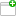This comment was attached:

arxcrash-2487-2097958164
#3
Comment posted byCrashBot
Dec 30, 05:46
A file was uploaded. cfg.iniThis comment was attached:

arxcrash-2487-2097958164
#4
Comment posted byCrashBot
Dec 30, 05:46
A file was uploaded. maps.txtThis comment was attached:

arxcrash-2487-2097958164
#5
Comment posted byCrashBot
Dec 30, 05:46
A file was uploaded. crash.txtThis comment was attached:

arxcrash-2487-2097958164